# Series and sequences

Find a fraction equivalent to the recurring decimal?

0.435643564356

n =  44
d =  101

### Step-by-step explanation:

$d=101=101$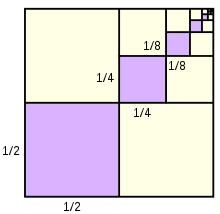Did you find an error or inaccuracy? Feel free to write us. Thank you!Tips to related online calculators
Need help to calculate sum, simplify or multiply fractions? Try our fraction calculator.

## Related math problems and questions:

• Decimal to fractionWrite decimal number 8.638333333 as a fraction A/B in the basic form. Given decimal has infinite repeating figures.
• Infinite decimalImagine the infinite decimal number 0.99999999 .. ... ... ... That is a decimal and her endless series of nines. Determine how much this number is less than the number 1. Thank you in advance.
• FractionFraction ? write as fraction a/b, a, b is integers numerator/denominator.
• Sum of seriesDetermine the 6-th member and the sum of a geometric series: 5-4/1+16/5-64/25+256/125-1024/625+....
• Fraction to decimal infinite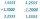Determine which digit is at 1000th place after the decimal point in the decimal expansion of the fraction 9/28.
• Infinite sum of areasAbove the height of the equilateral triangle ABC is constructed an equilateral triangle A1, B1, C1, of the height of the equilateral triangle built A2, B2, C2, and so on. The procedure is repeated continuously. What is the total sum of the areas of all tr
• Saving per cents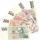The first day I save 1 cent and every next day cent more. How many I saved per year (365 days)?
• Sum 1-6Find the sum of the geometric progression 3, 15, 75,… to six terms.
• Sequences AP + GPThe three numbers that make up the arithmetic sequence have the sum of 30. If we subtract from the first 5, from the second 4 and keep the third, we get the geometric sequence. Find AP and GP members.
• Miraculous treeMiraculous tree grows so fast that the first day increases its height by half the total height of the second day by the third, the third day by a quarter, etc. How many times will increase its height after 6 days?
• Two geometric progressions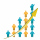Insert several numbers between numbers 6 and 384 so that they form with the given GP numbers and that the following applies: a) the sum of all numbers is 510 And for another GP to apply: b) the sum of entered numbers is -132 (These are two different geome
• Series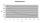Andrew wrote this series of numbers: 1,3,7,15, .... n. Which number in the series does not belong? ?
• InfinityIn a square with side 19 is inscribed circle, the circle is inscribed next square, again circle, and so on to infinity. Calculate the sum of the area of all these squares.
• Geometric progressionIn geometric progression, a1 = 7, q = 5. Find the condition for n to sum first n members is sn≤217.
• To the cinemaJane at 8 am got message that all 1093 school pupils will go to the cinema. Within 20 min she said it to the three friends. Each of them again for 20 minutes said to the other three. In this way the message spread further. At what time all the children in
• Sum of GP members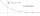Determine the sum of the GP 30, 6, 1.2, to 5 terms. What is the sum of all terms (to infinity)?
• Geometric progression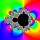Fill 4 numbers between 4 and -12500 to form geometric progression.